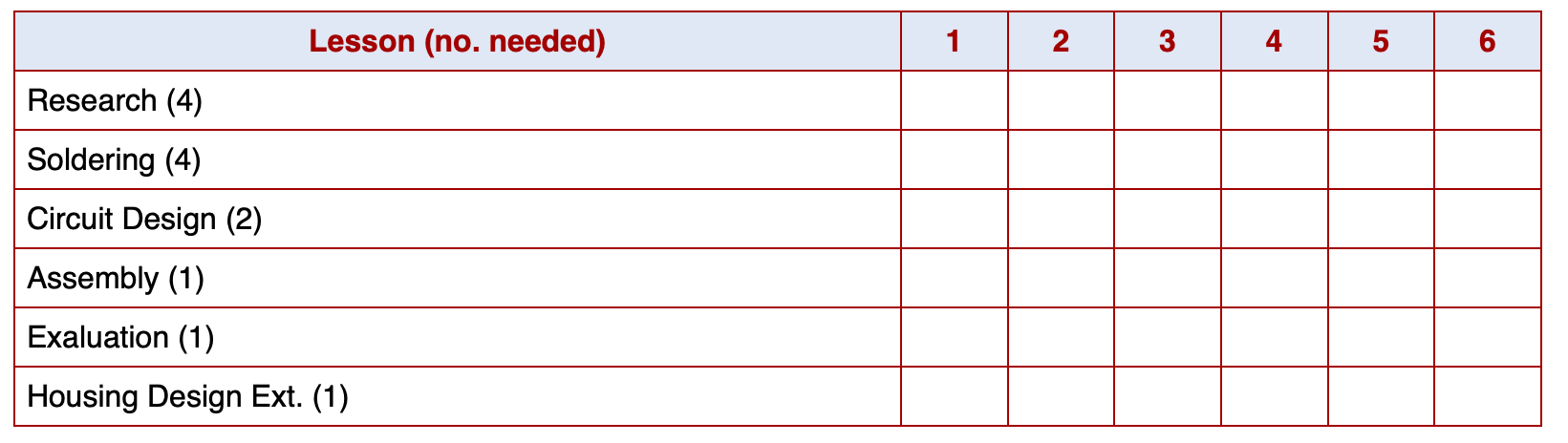# The LED Torch - Planning and Research

### Learn It

• When engineers have to complete large projects, they often rely on Gantt charts to help them organise themselves.
• A Gantt chart contains all the tasks you need to complete, and a date on which they will be completed.
• The Gantt chart below contains all the tasks for this project.• You can complete the tasks in any order you like, so long as they are all completed within the 6 lessons allowed.

### Try It

• Copy the table above and draw an `X` in the cell to indicate when you will complete each task.

## 2 Potential Difference

### Learn It

• Electricity is often described as a flow of electrons (tiny particles with a negative charge that you'll learn about in Science).
• Look at the animation below to see electrons flowing through a wire.

• Probably the most important concept you need to understand about the electricity is Potential Difference, which we often call the Voltage.
• Potential Difference is measured in Volts. Both the unit and the symbol for Voltage is a capital `V`.
• When a potential difference is applied to either ends of a wire (by using a battery or a power supply for instance), a force acts on electrons inside the wire.
• The force pulls the electrons towards the positive side of the battery or power supply.

### Try It

• Move the slider to increase and decrease the voltage acting across the wire.
• The best way to think about Voltage is to imagine it as the amount of energy each electron carries. This energy causes the electron to move, so the more energy it has, the faster it moves.
• If there is a voltage of 1 volt across a wire, each electron carries about 0.000000000016 Joules of energy. It takes about 1 Joule of energy to lift a small apple a metre into the air, so each electron carries a tiny amount of energy.

## 3 Assessment

#### Progress ladder - Yellow, Conduct some relevant research on a project, with assistance.

• In your own words, try to describe the effect on increasing and decreasing the voltage on the particles in the tube.

## 4 Current

### Learn It

• Another property of this flow of electrons is called Current.
• Writing down current is a little annoying. It's symbol is `I` but it is measured in Amps (`A`).
• Current is described as the rate of flow of electrons.

### Try It

• Move the slider to increase and decrease the voltage and note what happens to the current flowing in the wire..
• An easy way to think about current is to imagine how many electrons flow through a wire or a component every second.
• A current of 1A flowing through a wire means that there are 6240000000000000000 electrons flowing though it every second!

## 5 Assessment

#### Progress ladder - Green, Explain the terms voltage, current and resistance.

• In your own words, try to describe the relationship between the current flowing through the wire and the potential difference (voltage) across it.

## 6 Resistance

### Learn It

• The last property you need to be aware of is the Resistance of a component or a wire.
• As electrons pass through a wire or component they collide with atoms in the wire. This causes them lose a bit of their energy, and therefore we get a drop in Voltage (then energy carried by each electron)
• If a wire is very narrow, there will be more collisions so the resistance is higher.
• If a wire is very long, there will be more collisions so the resistance is higher.
• Some materials have arrangements of atoms inside them that means there are more collisions, so resistance is higher.

### Try It

• Move the slider to increase and decrease the resistance and note what happens to the current flowing in the wire..
• The easiest way to imagine resistance is the narrowing of the wire. If you were to imagine water flowing through a pipe, it would find it more difficult to get through a narrower pipe than a wide one.
• Resistance has the symbol `R` and is measured in Ohms (`Ω`)